# Replace values from dataframe column using R

In this article, we will discuss how to replace values from a DataFrame column in R Programming Language.

Whenever we deal with some data, it is necessary that we draw accurate conclusions from it. Now imagine what if, there are some missing values(this happens when some observation is missing in a column of a data-frame or contains a character value instead of a numerical value) in our data or let’s say there are some negative values in the dataset, which might lead to false accuracy, also it affects the analysis result. So, to avoid these, we can replace such values, using the following methods.

Example 1. Replacing NA values in a data frame with Zeroes(0’s)

So first, we create a table with the column names: Name, ID, CPI and add respective values to the respective columns

## R

 `Name <- ``c``(``"Amy"``, ``"Celine"``, ``"Lily"``, ` `          ``"Irene"``, ``"Rosy"``, ``"Tom"``, ``"Kite"``) ` `ID <- ``c``(123, ``NA``, 134, ``NA``, 166, 129, 178) ` `CPI <- ``c``(8.5, 8.3, 7.8, ``NA``, 6.9, 9.1, 5.6) ` ` `  `details <- ``data.frame``(Name, ID, CPI) ` `View``(details)`

Output: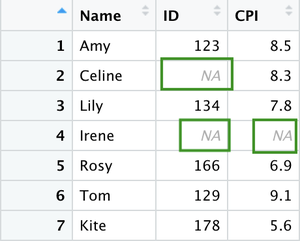Replace value from NA values to 0:

## R

 `details[``is.na``(details)] <- 0 ` ` `  `# views the newly modified table ` `View``(details)`

Output: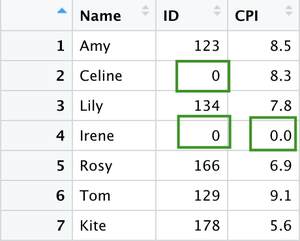Example 2. Replacing NA values in a data frame with the mean values

We create the table with the following columns and values, same as explained before.

## R

 `RollNo <- ``c``(24,23,``NA``,18) ` `ID <- ``c``(123, 156, 134, 148) ` `CPI <- ``c``(8.5,8.3,7.8,``NA``) ` ` `  `secR1 <- ``data.frame``(RollNo,ID,CPI) ` `View``(secR1)`

Output: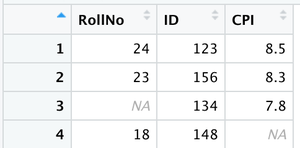Table

Now replacing the NA value with the mean value:

## R

 `ex <- secR1 ` `ex ` ` `  `# replacing the NA value with the mean value ` `ex\$RollNo[``is.na``(ex\$RollNo)]<-``mean``(ex\$RollNo, na.rm = T) ` `round``(ex, digits = 0)`

Output: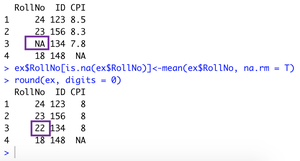The Output –> NA in Roll no. replaced with the mean

Example 3. Replacing the negative values with 0s(zeroes):

First, we create a table, as we did before.

## R

 `RollNo <- ``c``(24,23,16,-18) ` `ID <- ``c``(123,156,-134,148) ` `CPI <- ``c``(8.5,8.3,7.8,8.9) ` `marks <- ``c``(-54,70,-20,9) ` ` `  `secR1 <- ``data.frame``(RollNo,ID,CPI,marks) ` `View``(secR1)`

Output: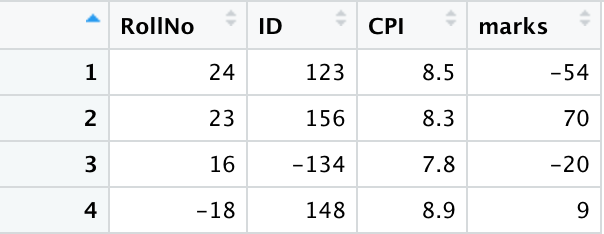Now change the negative values to 0:

## R

 `secR1[secR1 < 0] <- 0      ` `view``(secR1)`

Output: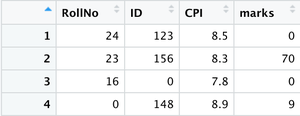Example 4. Using replace( ) method:

The replace() function, replaces the values in x with indices given in the list by those given in values. If necessary, the values in values are recycled.

Syntax: replace(x, list, values)

Arguments:

• x: vector
• list: an index vector
• values: replacement values

Example:

## R

 `# creates a table ` `RollNo <- ``c``(24,23,``NA``,18) ` `ID <- ``c``(123, 156, 134, 148) ` `CPI <- ``c``(8.5,8.3,7.8,``NA``) ` `secR1 <- ``data.frame``(RollNo,ID,CPI)    ` `View``(secR1)   ` ` `  `# 2nd element of the list, hence 2, replacement value is 45 ` `secR1<- ``replace``(RollNo, 2, 45)      ` `View``(secR1)`

Output: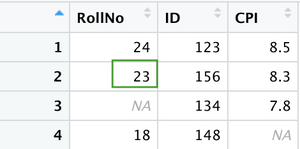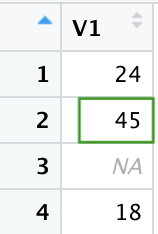Final Output

Whether you're preparing for your first job interview or aiming to upskill in this ever-evolving tech landscape, GeeksforGeeks Courses are your key to success. We provide top-quality content at affordable prices, all geared towards accelerating your growth in a time-bound manner. Join the millions we've already empowered, and we're here to do the same for you. Don't miss out - check it out now!

Previous
Next